# Theorems of Continuity JEE Notes | EduRev

## JEE : Theorems of Continuity JEE Notes | EduRev

The document Theorems of Continuity JEE Notes | EduRev is a part of the JEE Course Mathematics (Maths) Class 12.
All you need of JEE at this link: JEE

C. Theorems of Continuity

THEOREM–1 If f & g are two functions that are continuous at x= c then the functions defined by F1(x) = f(x) ± g(x) ; F2(x) = K f(x)  K any real number ; F3(x) = f(x).g(x) are also continuous at x= c.
Further, if g (c) is not zero, then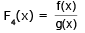is also continuous at x = c.

THEOREM–2 If  f(x) is continuous & g(x) is discontinuous at x = a  then the product function ø(x) = f(x) . g(x) is not necessarily discontinuous at x = a .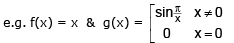THEOREM–3 If f(x) and g(x)  both  are  discontinuous  at  x = a  then  the  product  function ø(x) = f(x) . g(x) is not necessarily discontinuous at  x = a .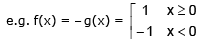Theorems-4 : Intermediate Value Theorem

If f is continuous on the closed interval [a, b] and k is any number between f(a) and f(b), then there is at least one number c in [a, b] such that f(c) = k.

Note that the Intermediate Value Theorem tells that at least one c exists, but it does not give a method for finding c. Such theorems are called existence theorems.

As a simple example of this theorem, consider a person's height. Suppose that a girl is 5 feet tall on her thirteenth birthday and 5 feet 7 inches tall on her fourteenth birthday. Then, for any height h between 5 feet and 7 inches, there must have been a time t when her height was exactly h. This seems reasonable because human growth is continuous and a person's height does not abruptly change from one value to another.

The Intermediate Value Theorem guarantees the existence of at least one number c in the closed interval [a, b]. There may, of course, be more than one number c such that f(c) = k, as shown in Figure 1. A function that is not continuous does not necessarily possess the intermediate value property. For example, the graph of the function shown in Figure 2 jumps over the horizontal line given by y = k and for this function there is no value of c in [a, b] such that f(c) = k.

The Intermediate Value Theorem often can be used to locate the zeroes of a function that is continuous on a closed interval. Specifically, if f is continuous on [a, b] and f(a) and f(b) differ in sign, then the intermediate Value Theorem guarantees the existence of at least one zero of f in the closed interval [a, b].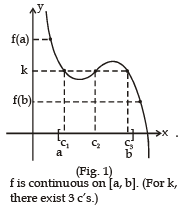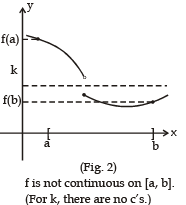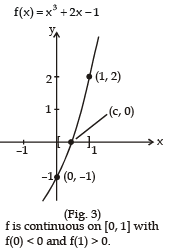Ex.10 Use the Intermediate Value Theorem to show that the polynomial function f(x) = x3 + 2x - 1 has a zero in the interval [0, 1]

Sol. Note that f is continuous on the closed interval [0, 1]. Because

f(0) = 03 + 2(0) - 1 = -1         and                f(1) = 13 + 2(1) - 1 = 2

it follows that f(0) < 0 and f(1) > 0. You can therefore apply the Intermediate Value Theorem to conclude that there must be some c in [0, 1] such that f(c) = 0, as shown in Figure 3.

Ex.11 State intermediate value theorem and use it to prove that the equation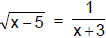has at least one real root.

Sol. Let f (x) =first, f (x) is continuous on [5, 6]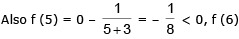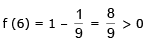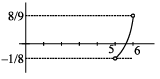Hence by intermediate value theorem É at least one value of c ∈ (5, 6) for which f (c) = 0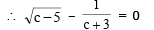c is root of the equation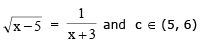Ex.12 If f(x) be a continuous function in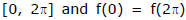then prove that there exists point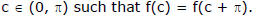Sol.

Let g(x) = f(x) – f(x + π) ....(i)

at x = π; g(π) = f(π) – f(2π) ....(ii)

at x = 0, g(0) = f(0) – f(π) ...(iii)

adding (ii) and (iii), g(0) + g(π) = f(0) – f(2π)

⇒ g(0) + g(π) = 0 [Given f(0) = f(2π) ⇒ g(0) = –g(π)

⇒ g(0) and g(π) are opposite in sign.

⇒ There exists a point c between 0 and p such g(c) = 0 as shown in graph;

From (i) putting x = c g(c) = f(c) – f(c +π) = 0 Hence, f(c) = f(c + π)

Offer running on EduRev: Apply code STAYHOME200 to get INR 200 off on our premium plan EduRev Infinity!

## Mathematics (Maths) Class 12

209 videos|222 docs|124 tests

,

,

,

,

,

,

,

,

,

,

,

,

,

,

,

,

,

,

,

,

,

;